# Find u. (v * w). This quantity is called the triple scalar product of u, v,...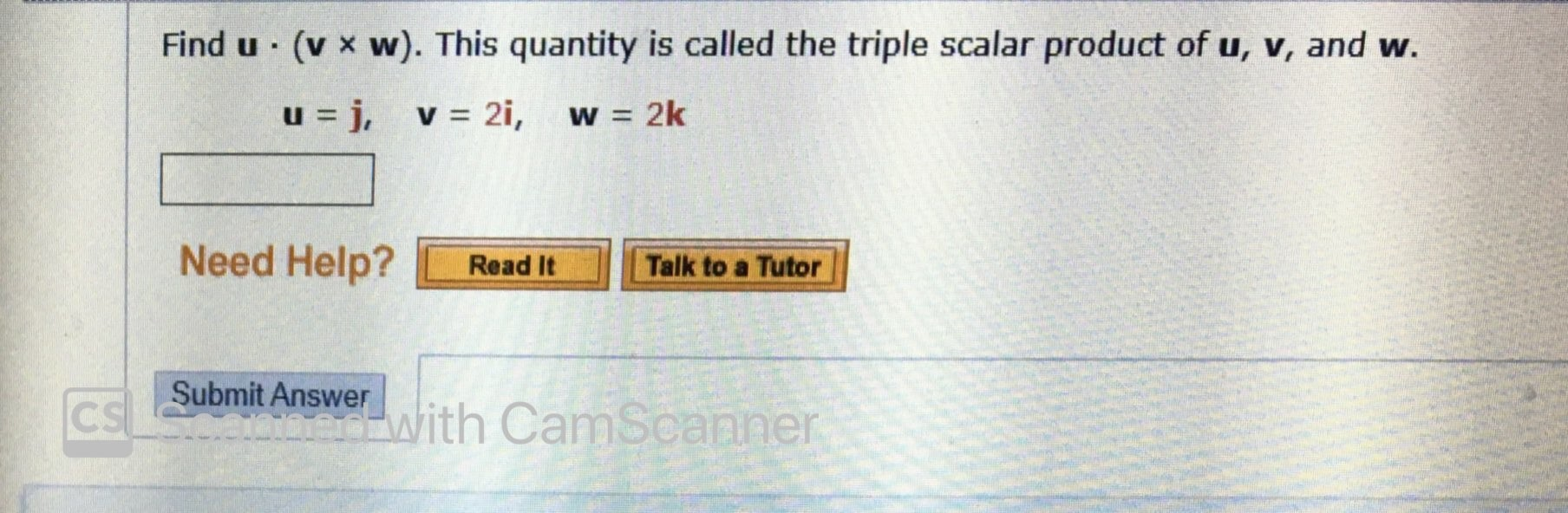Find u. (v * w). This quantity is called the triple scalar product of u, v, and w. u=j, v = 2i, w = 2k Need Help? Read It Talk to a Tutor CS Submit Answer With CamScanner onit Answer with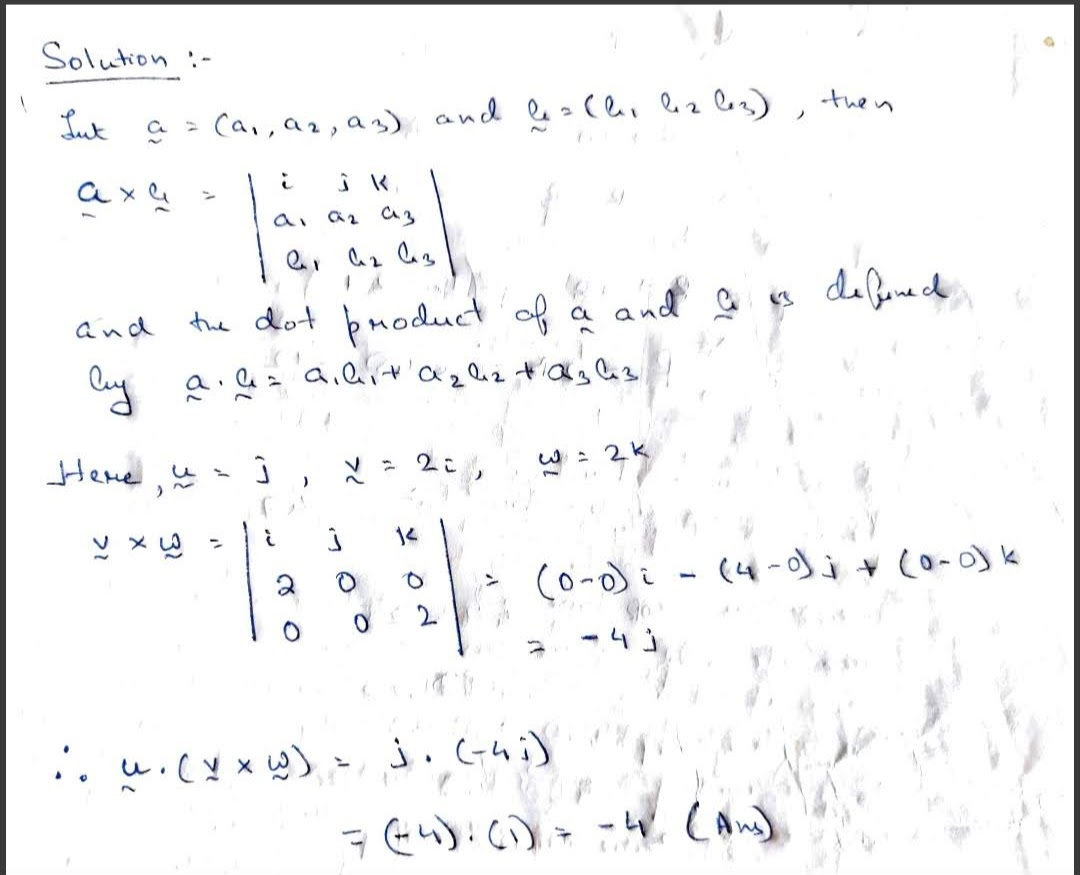##### Add Answer of: Find u. (v * w). This quantity is called the triple scalar product of u, v,...
Similar Homework Help Questions
• ### Thank you Find u. (w). This quantity is called the triple scalar product of u, v,...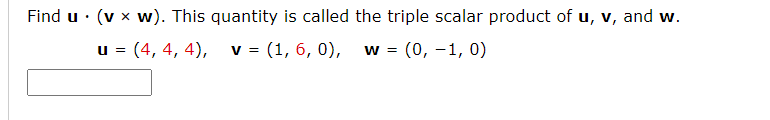Thank you Find u. (w). This quantity is called the triple scalar product of u, v, and w. u = (4, 4, 4), v = (1, 6, 0), (0, -1,0) W = Let T: R3 R3 be a linear transformation such that T(1, 1, 1) = (4,0, -1), T(0, -1, 2) = (-5,2, -1), and T(1, 0, 1) = (1, 1, 0). Find the indicated image. T(2, -1, 1) T(2, -1, 1) = Let T be a linear transformation from...

• ### QUESTION 10 Find the triple scalar product (u x v). w of the vectors u =...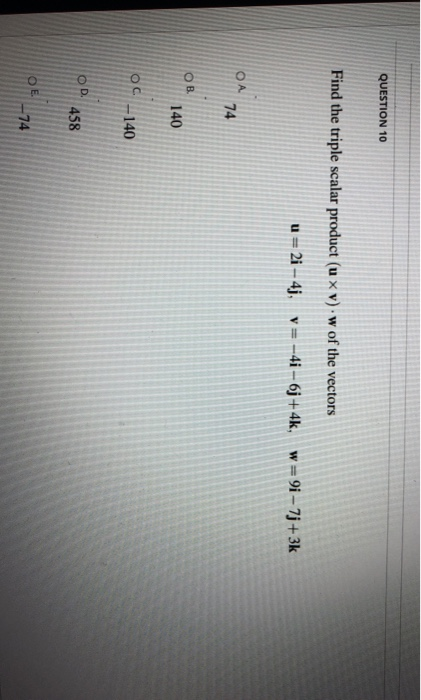QUESTION 10 Find the triple scalar product (u x v). w of the vectors u = 2i - 4j, v= -4i - 6j + 4k, w=9i - 7j+3k ОА 74 OB 140 OC-140 OD 458 ОЕ 74

• ### Find a unit vector orthogonal to both u and v. u = i - 2j V...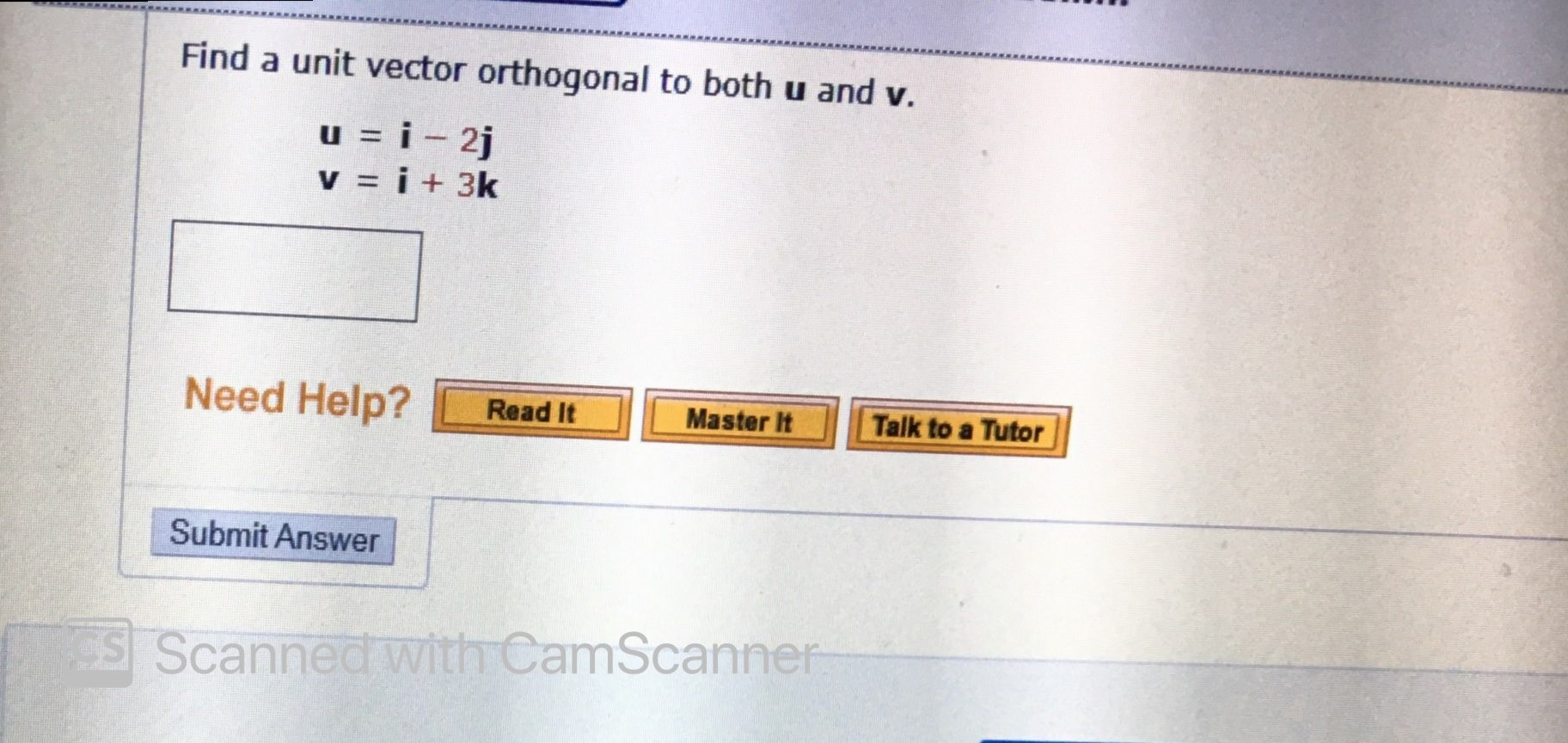Find a unit vector orthogonal to both u and v. u = i - 2j V = i + 3k Need Help? Read It Master It Talk to a Tutor Submit Answer Scanned with CamScanner

• ### TETETTERE Find u xv. u = (0, 1, -6), v= (1, -1,0) Show that u x...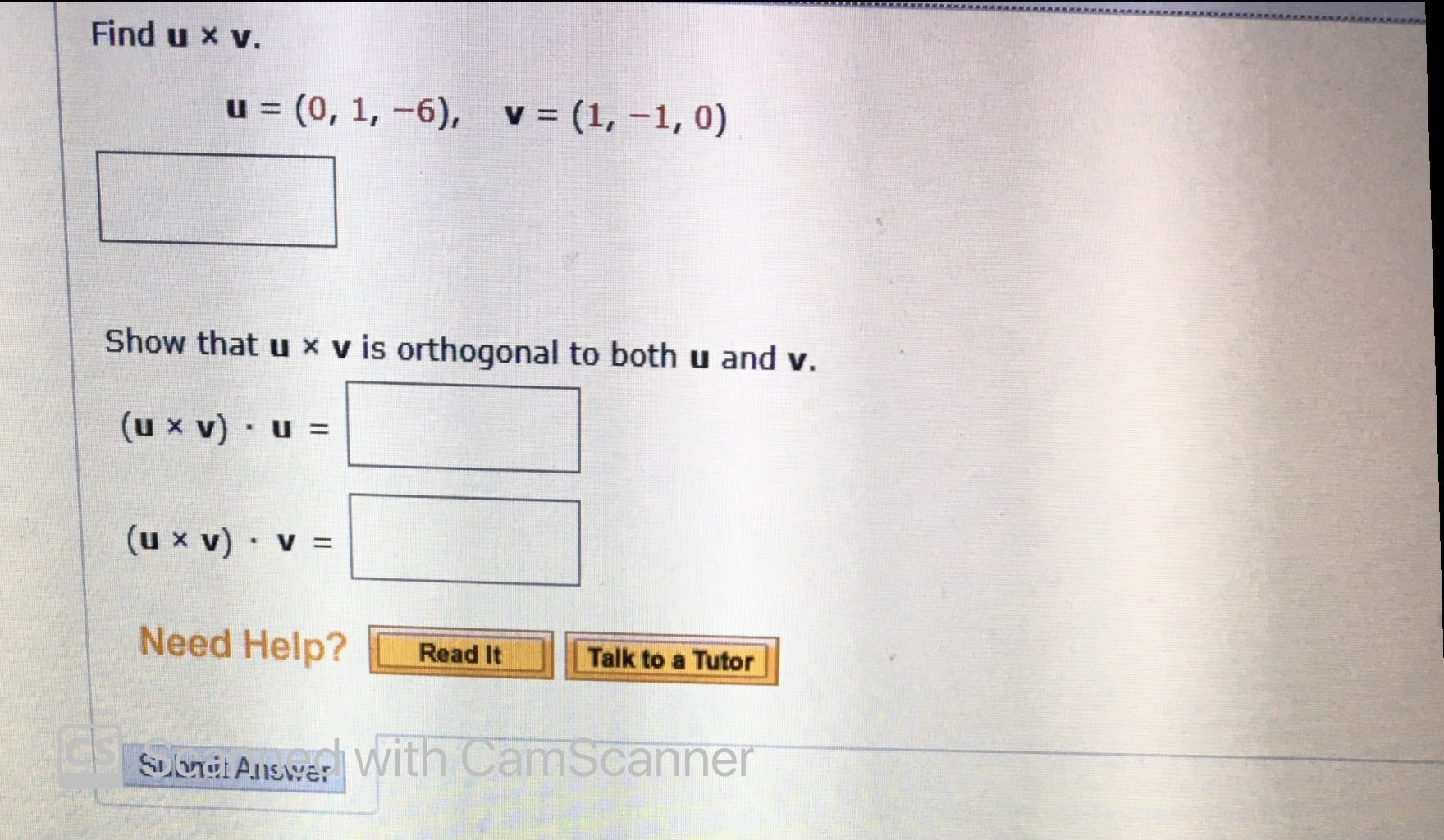TETETTERE Find u xv. u = (0, 1, -6), v= (1, -1,0) Show that u x v is orthogonal to both u and v. (u X v) · u = (u x v) v = Need Help? Read It Talk to a Tutor Solond Answer with CamScanner

• ### 4. Determine the scalar triple product of the vectors: 3i2k and c = 3i+ j +...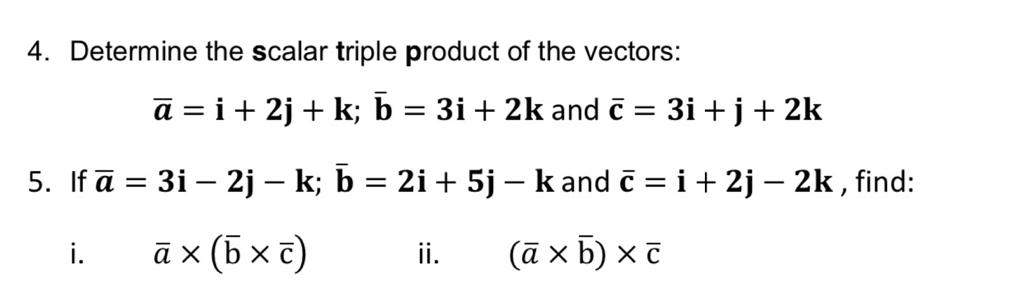4. Determine the scalar triple product of the vectors: 3i2k and c = 3i+ j + 2k a i2jk; b 5. Ifa 3i 2j - k; b = 2i +5j - kand C i + 2j - 2k, find: ax (b x c) (ax b) x t i. ii 4. Determine the scalar triple product of the vectors: 3i2k and c = 3i+ j + 2k a i2jk; b 5. Ifa 3i 2j - k; b = 2i +5j -...

• ### Calculate u v wPlease help with this one!!!!Let u = 3i-j-2k, v = -2i-3j+2k, and w = i+2kcalculate

• ### scalar products lifesaver

use scalar triple product to verify thats these vectors arecoplaneru=i+5j-2k, v=3i-j and w=5i+9j-4k

• ### Triple scalar productGive: vector u = 2i +jvectorv = 2i - j + kvectorw = i + 2kVerify that(u x v) w=(v x w) u=(w x u) vand find the volume of the parallelepiped (box) determined byvectors u, v, and w.

• ### Find u xv, vxu, and vxv. u = i- j, v=j+k (a) U XV -i-j+k x...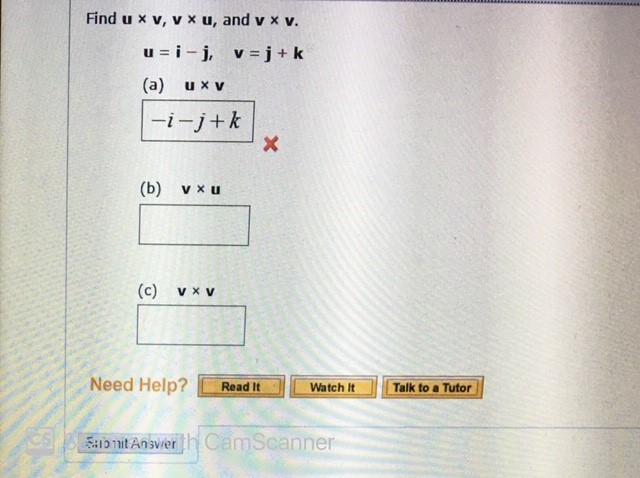Find u xv, vxu, and vxv. u = i- j, v=j+k (a) U XV -i-j+k x (b) V XU (c) V XV Need Help? Read It Watch It Talk to a Tutor OS Zato nitansver th Cam Scanner

• ### Chapter 13 Section 13.4 The Cross Product #37

Use the scalar triple product to verify that the vectors u = i + 5j - 2k, v = 3i - j, and w = 5i + 9j - 4k are coplanar

Free Homework App